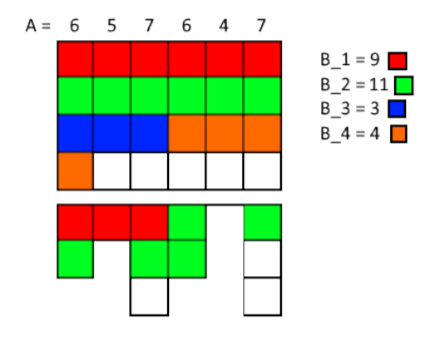# Baltic Olympiad in Informatics[BOI2019]Tom’s Kitchen

### Tom’s Kitchen

Tom’s Kitchen 1 sec / 10 sec 256 MB
Tom’s Kitchen is a very popular restaurant. One of the reasons for its popularity is that every
single meal is prepared by at least K different chefs. Today, there are N meals to be prepared,
with meal i needing Ai hours of work.
There are M chefs which Tom can hire to prepare all the meals but the chef j will work at most
Bj hours. Additionally, even when he works less, he still wants to be paid for the full Bj hours.
A chef can work on several meals for different amounts of time, but any meal will be properly
prepared only if at least K chefs take part in preparing it and the total time they spend is
exactly Ai
. When a chef takes part in preparing a meal, he always works on it some positive
integer number of hours.
Tom needs help in choosing the optimal subset of chefs such that the sum of hours where the
chefs are getting paid without working is minimized.
Input. The first line contains the integers N, M, and K.
The second line contains N integers Ai and the third line M integers Bj .
Output. The only line should contain the number of hours the chefs spend not working but
still getting paid when Tom chooses the optimal subset to hire. If there is no way to prepare all
the N meals according to the rules described above, output “Impossible”.
Example. Input
1 2 2
5
3 4
Output
2
Here Tom needs two chefs to work on the meal, so he must hire both that are available. Then
it does not matter how they divide the work, as they end up working a total of 5 hours, but
getting paid for 3 + 4 = 7 hours, and thus getting paid for 2 extra hours.
Example. Input
1 1 2
5
5
Output
Impossible
Here Tom needs two chefs to work on the meal, but only one is available.
Example. Input
3 3 3
3 3 2
3 3 3
Output
Impossible
Here meal 3 can’t be prepared by three chefs, as each would have to work for at least an hour,
but the meal takes only 2 hours to prepare.
Grading. The test groups satisfy the following conditions:

1. (9 points) 1 ≤ N, K ≤ 300, 1 ≤ M ≤ 2, 1 ≤ Ai, Bj ≤ 300.
2. (22 points) 1 ≤ N, K ≤ 300, 1 ≤ M ≤ 15, 1 ≤ Ai, Bj ≤ 300.
3. (20 points) 1 ≤ N, M, Ai, Bj ≤ 300, K = 1.
4. (21 points) 1 ≤ N, M, K, Ai, Bj ≤ 40.
5. (28 points) 1 ≤ N, M, K, Ai, Bj ≤ 300. 7

### 题目大意

n n 块肉，第 i i 块有 A i A_i 斤，有 m m 个厨师，第 i i 个厨师最多工作 B i B_i 个小时，而且没小时只能处理1斤肉，每一块肉至少需要 k k 个厨师参与处理，现在雇佣一些厨师使得他们的摸鱼时间(不工作时间)之和最少。

### 题解d p [ i ] dp[i] 表示总工作为i的前提下，在猫排工作的最大时间，那么
d p [ i + b ] = m a x ( d p [ i + b ] , d p [ i ] + m i n ( b , n ) ) ; dp[i+b]=max(dp[i+b],dp[i]+min(b,n));

#include<cstdio>
#include<cstring>
#include<queue>
#include<algorithm>
using namespace std;
const int MAXN=305,MAXM=90000;
int n,m,k,ans,jl=0;
int dp[MAXN*MAXN];
int main()
{
freopen("kitchen.in","r",stdin);
freopen("kitchen.out","w",stdout);
scanf("%d %d %d",&n,&m,&k);
for(int i=1;i<=n;i++)
{
int a;
scanf("%d",&a);
if(a<k)
{
printf("Impossible\n");
return 0;
}
jl+=a;
}
memset(dp,-1,sizeof(dp));
dp=0;
for(int i=1;i<=m;i++)
{
int a,b;
scanf("%d",&b);
a=min(n,b);
for(int j=MAXM-b;j>=0;j--)
{
if(dp[j]!=-1)
{
dp[j+b]=max(dp[j+b],dp[j]+a);
}
}
}
for(int i=jl;i<=MAXM;i++)
{
if(dp[i]>=n*k)
{
printf("%d\n",i-jl);
return 0;
}
}
printf("Impossible\n");
fclose(stdin);
fclose(stdout);
}



10-24
07-27256
09-07591
06-18119
07-04
02-183034
02-14966
02-12464
11-07283
09-25271
09-23138
09-20
09-20
09-20
09-20

### “相关推荐”对你有帮助么？

•非常没帮助
•没帮助
•一般
•有帮助
•非常有帮助被折叠的  条评论 为什么被折叠?到【灌水乐园】发言jyzryz

¥1 ¥2 ¥4 ¥6 ¥10 ¥20获取中扫码支付点击重新获取扫码支付1.余额是钱包充值的虚拟货币，按照1:1的比例进行支付金额的抵扣。
2.余额无法直接购买下载，可以购买VIP、付费专栏及课程。余额充值